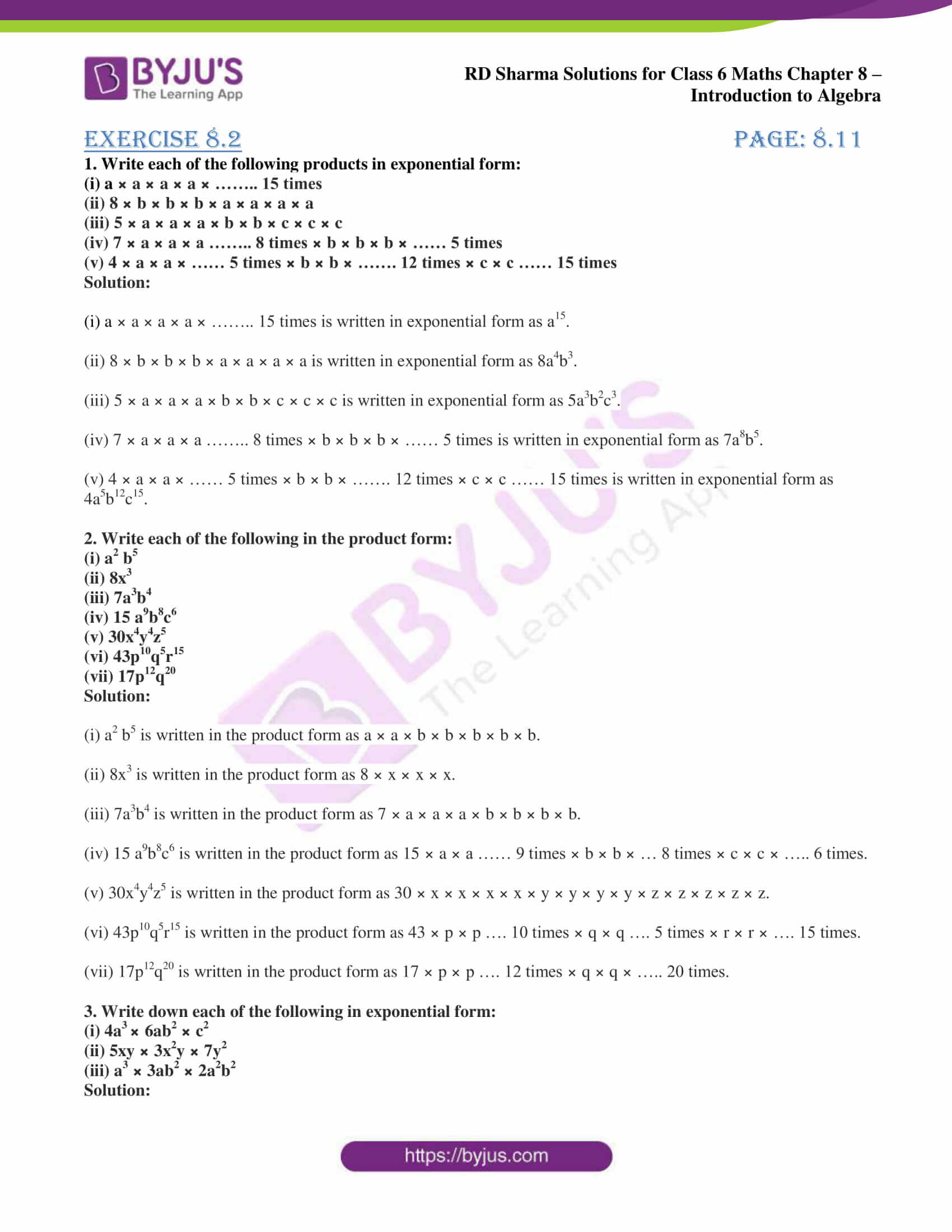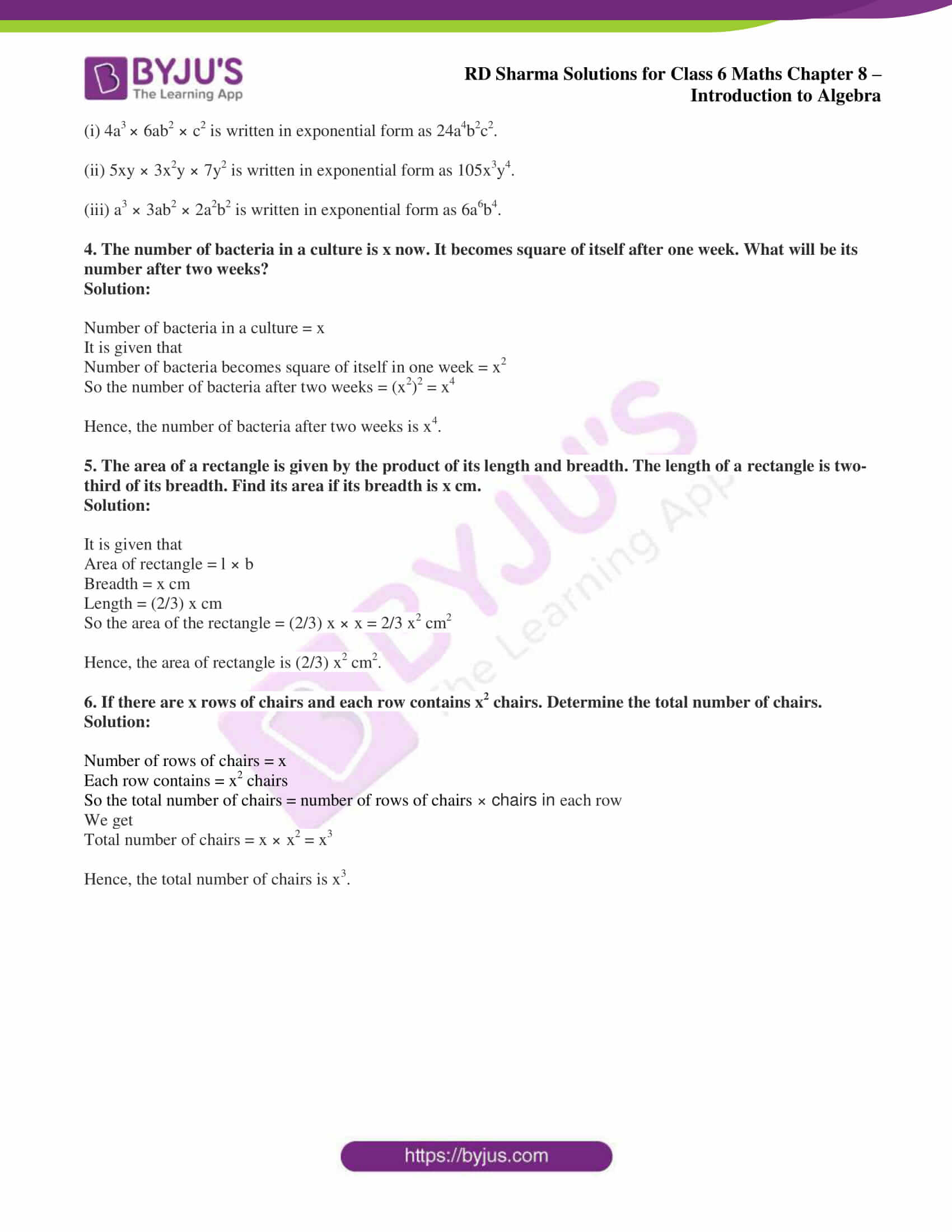# RD Sharma Solutions for Class 6 Maths Chapter 8: Introduction to Algebra Exercise 8.2

The students who aspire to perform well in the exam can solve problems using RD Sharma Solutions Class 6. The solutions consist of explanations in a simple language which matches the understanding ability of students. The problems of RD Sharma textbook can be solved by using the solutions as a reference guide. With the help of RD Sharma Solutions for Class 6 Maths Chapter 8 Introduction to Algebra Exercise 8.2 PDF students can expertise the concepts which are important for the exam preparation.

## RD Sharma Solutions for Class 6 Maths Chapter 8: Introduction to Algebra Exercise 8.2 Download PDF### Access answers to Maths RD Sharma Solutions for Class 6 Chapter 8: Introduction to Algebra Exercise 8.2

1. Write each of the following products in exponential form:

(i) a × a × a × a × …….. 15 times

(ii) 8 × b × b × b × a × a × a × a

(iii) 5 × a × a × a × b × b × c × c × c

(iv) 7 × a × a × a …….. 8 times × b × b × b × …… 5 times

(v) 4 × a × a × …… 5 times × b × b × ……. 12 times × c × c …… 15 times

Solution:

(i) a × a × a × a × …….. 15 times is written in exponential form as a15.

(ii) 8 × b × b × b × a × a × a × a is written in exponential form as 8a4b3.

(iii) 5 × a × a × a × b × b × c × c × c is written in exponential form as 5a3b2c3.

(iv) 7 × a × a × a …….. 8 times × b × b × b × …… 5 times is written in exponential form as 7a8b5.

(v) 4 × a × a × …… 5 times × b × b × ……. 12 times × c × c …… 15 times is written in exponential form as 4a5b12c15.

2. Write each of the following in the product form:

(i) a2 b5

(ii) 8x3

(iii) 7a3b4

(iv) 15 a9b8c6

(v) 30x4y4z5

(vi) 43p10q5r15

(vii) 17p12q20

Solution:

(i) a2 b5 is written in the product form as a × a × b × b × b × b × b.

(ii) 8x3 is written in the product form as 8 × x × x × x.

(iii) 7a3b4 is written in the product form as 7 × a × a × a × b × b × b × b.

(iv) 15 a9b8c6 is written in the product form as 15 × a × a …… 9 times × b × b × … 8 times × c × c × ….. 6 times.

(v) 30x4y4z5 is written in the product form as 30 × x × x × x × x × y × y × y × y × z × z × z × z × z.

(vi) 43p10q5r15 is written in the product form as 43 × p × p …. 10 times × q × q …. 5 times × r × r × …. 15 times.

(vii) 17p12q20 is written in the product form as 17 × p × p …. 12 times × q × q × ….. 20 times.

3. Write down each of the following in exponential form:

(i) 4a3 × 6ab2 × c2

(ii) 5xy × 3x2y × 7y2

(iii) a3 × 3ab2 × 2a2b2

Solution:

(i) 4a3 × 6ab2 × c2 is written in exponential form as 24a4b2c2.

(ii) 5xy × 3x2y × 7y2 is written in exponential form as 105x3y4.

(iii) a3 × 3ab2 × 2a2b2 is written in exponential form as 6a6b4.

4. The number of bacteria in a culture is x now. It becomes square of itself after one week. What will be its number after two weeks?

Solution:

Number of bacteria in a culture = x

It is given that

Number of bacteria becomes square of itself in one week = x2

So the number of bacteria after two weeks = (x2)2 = x4

Hence, the number of bacteria after two weeks is x4.

5. The area of a rectangle is given by the product of its length and breadth. The length of a rectangle is two-third of its breadth. Find its area if its breadth is x cm.

Solution:

It is given that

Area of rectangle = l × b

Length = (2/3) x cm

So the area of the rectangle = (2/3) x × x = 2/3 x2 cm2

Hence, the area of rectangle is (2/3) x2 cm2.

6. If there are x rows of chairs and each row contains x2 chairs. Determine the total number of chairs.

Solution:

Number of rows of chairs = x

Each row contains = x2 chairs

So the total number of chairs = number of rows of chairs × chairs in each row

We get

Total number of chairs = x × x2 = x3

Hence, the total number of chairs is x3.

#### 1 Comment

1. Magathi

Amazing app. 100/100